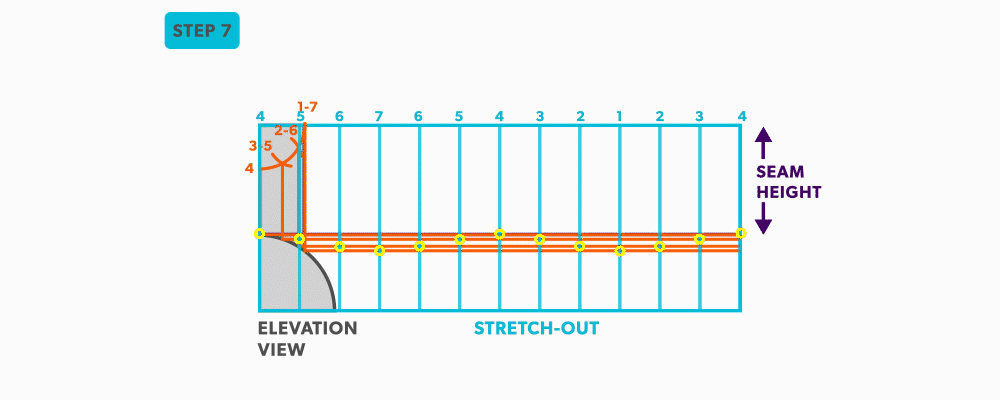Parallel Line Pattern Development

# 16 Unequal Diameter 90 Degree Tee-Shop Method

We will continue to use short-cuts. In this example, we move the views into the blank size and minimize our drawing. It still gives us the point “where the tee hits the pipe” and it is much more manageable to do in the shop. This method can be used on any tee besides an oblique tee.
1. Calculate the blank size. Use the stretch-out for one dimension () and the seam height plus the radius of the pipe for the second (vertical) dimension. Add any seam allowances on before shearing the blank size.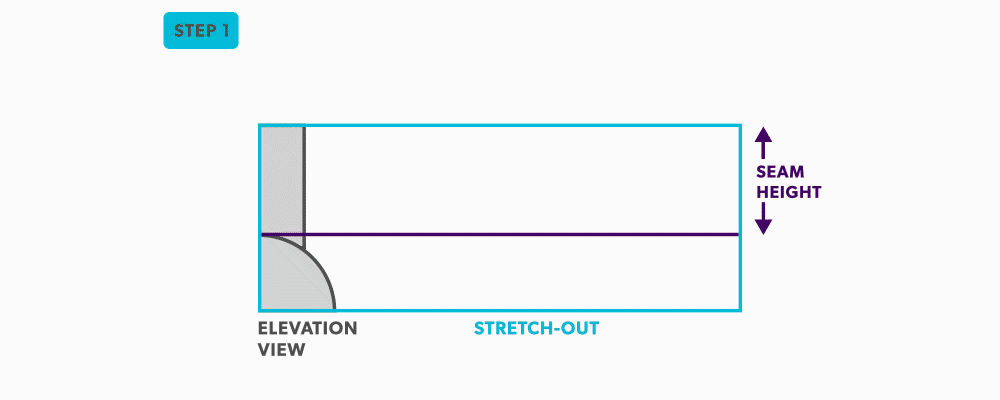2. With your dividers, swing the of the pipe to 180° at the bottom of the blank. Then, from the same radius point, swing the radius of the tee and divide it into 6 equal parts (see Divide a Circle Into 12 Equal Parts) and label it. Remember that only the tee is divided. Also, notice that the half circles are symmetrical, so a quarter circle is the minimum required in this case.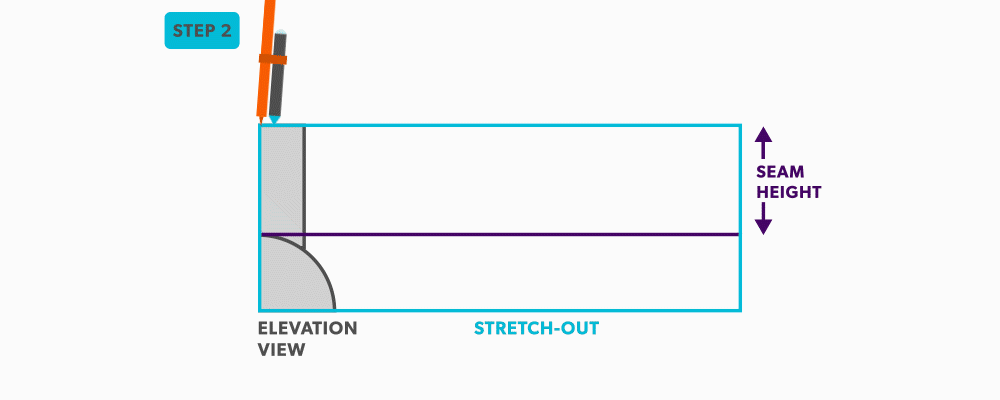3. Project the divisions of the tee into the pipe and where they intersect, project them horizontally.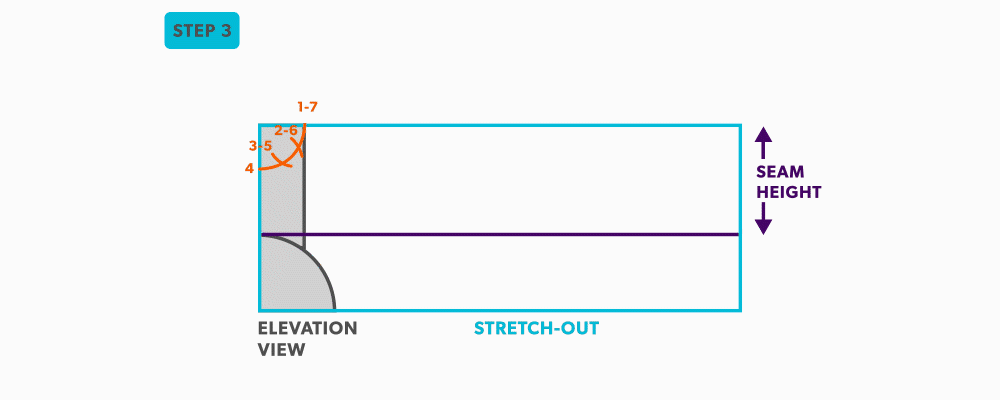4. Divide the blank size into 12 equal parts (see Divide a Line Using a Ruler on an Angle). Remember to only divide the circumference, any seam allowance should not be included in the divisions.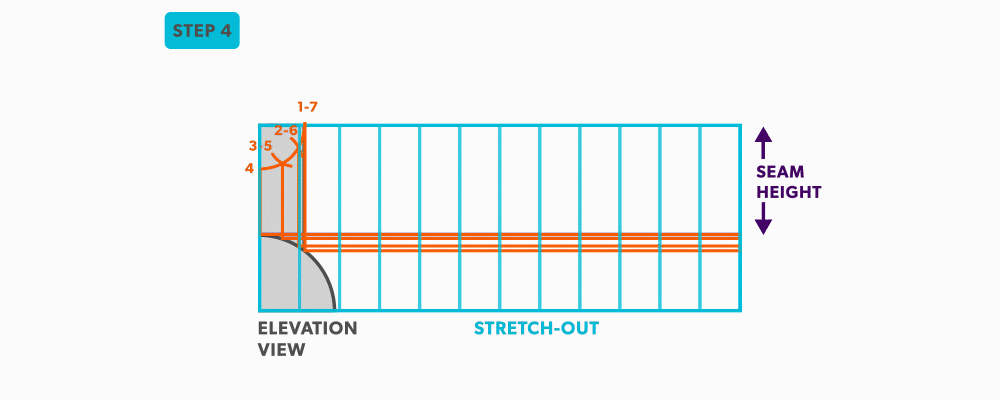5. Label the to match the , starting at the seam.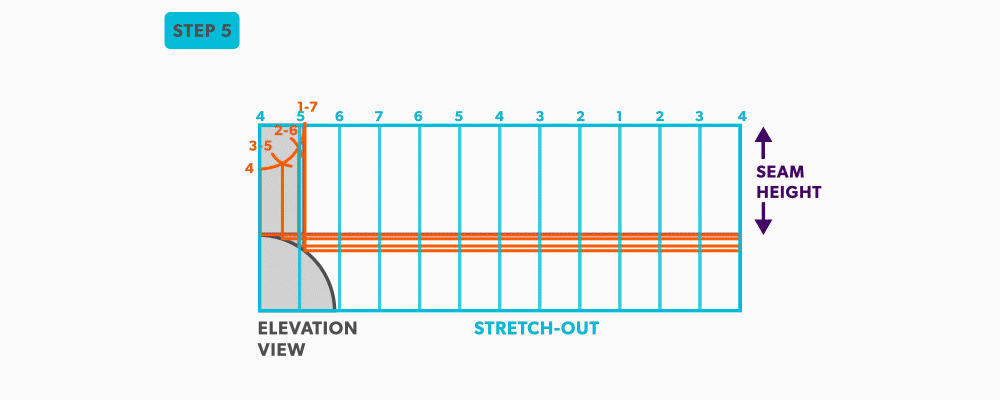6. Start the at your seam and follow the labeling. Notice the pattern of over 1, up/down 1, until you reach the last line and then it reverses.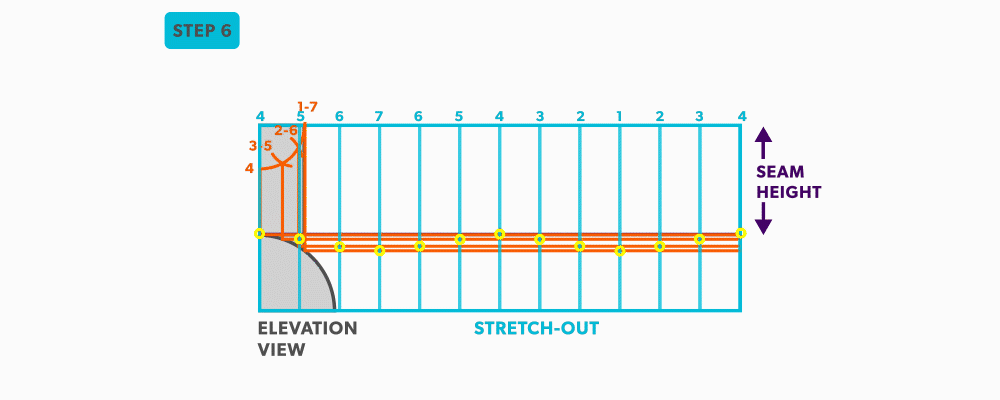7. Draw the line on the pattern with a flexible curve